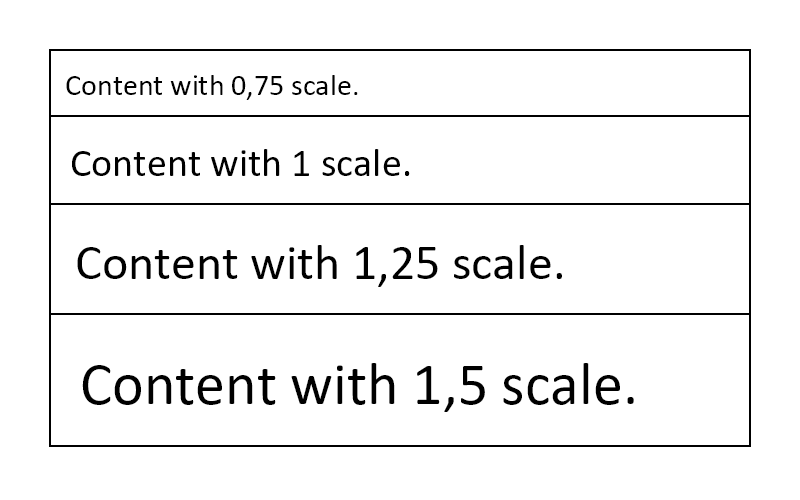# Scale #

• This component scales the size of its children,
• The layout rules, such as available space is being scaled as well so the container follow all size constraints.
csharp
``````.Scale(2f)

.ScaleHorizontal(2f)
.ScaleVertical(2f)
``````
``````.Scale(2f)

.ScaleHorizontal(2f)
.ScaleVertical(2f)
``````

Example:

csharp
``````.Column(column =>
{
var scales = new[] { 0.75f, 1f, 1.25f, 1.5f };

foreach (var scale in scales)
{
column
.Item()
.Border(1)
.Scale(scale)
.Text(\$"Content with {scale} scale.")
.FontSize(20);
}
});
``````
``````.Column(column =>
{
var scales = new[] { 0.75f, 1f, 1.25f, 1.5f };

foreach (var scale in scales)
{
column
.Item()
.Border(1)
.Scale(scale)